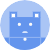search
Search
Publish
Guest 0reps
Thanks for the thanks!
close
account_circle
Profile
exit_to_app
Sign out
search
keyboard_voice
close
Searching Tips
Search for a recipe: "Creating a table in MySQL"
Search for an API documentation: "@append"
Search for code: "!dataframe"
Apply a tag filter: "#python"
Useful Shortcuts
/ to open search panel
Esc to close search panel
to navigate between search results
d to clear all current filters
Enter to expand content previewDoc SearchCode Search BetaSORRY NOTHING FOUND!
mic
Start speaking...Voice search is only supported in Safari and Chrome.
Shrink
Navigate to
A
A
share
thumb_up_alt
bookmark
arrow_backShareTwitterFacebook

# NumPy | var method

Programming
chevron_right
Python
chevron_right
NumPy
chevron_right
Documentation
schedule Mar 10, 2022
Last updated
local_offer PythonNumPy
Tags

NumPy's var(~) method computes the variance of values in the input array. The variance is computed using the following formula:

$$\frac{1}{N}\sum_{i=0}^{N}\left(x_i-\bar{x}^2\right)$$

Where:

• $N$ is the size of the given array (i.e. the sample size)

• $x_i$ is the value of the $i$th index in the Numpy array

• $\bar{x}$ is the sample mean

NOTE

var(~) method can also compute the unbiased estimate of the variance. We do this by setting ddof=1 in the parameters, as we shall see later in the examples.

# Parameters

1. a | array-like

The array on which to perform the method.

2. axislink | int or tuple | optional

The axis along which we compute the variance. For 2D arrays, the allowed values are as follows:

Axis

Meaning

0

Variance will be computed column-wise

1

Variance will be computed row-wise

None

Variance will be computed on a flattened array

By default, axis=None.

3. dtype | string or type | optional

The type used to compute the variance. If the input array is of type int, then float32 will be used. If the input array is of another numerical type, then its type will be used.

4. ddoflink | int | optional

The delta degree of freedom. This can be used to modify the denominator in the front:

$$\frac{1}{N\color{blue}{-ddof}}\sum_{i=0}^{N}\left(x_i-\bar{x}^2\right)$$

By default, ddof=0.

# Return value

If axis=None, then a single float representing the variance of all the values in the array is returned. Otherwise, a Numpy array is returned.

# Examples

## Variance of a 1D array

 np.var([1,2,3,4]) 1.25 

## Computing sample variance

To compute the sample variance, set ddof=1:

 np.var([1,2,3,4], ddof=1) 1.6666666666666667 

## Computing population variance

To compute the population variance, leave out the ddof parameter or explicitly set ddof=0:

 np.var([1,2,3,4])   # By default, ddof=0 1.25 

## Variance of a 2D array

### Entire array

Without specifying the axis parameter, Numpy will just regard your Numpy array as a flattened array.

 np.var([[1,2],[3,4]]) 1.25 

This code is fundamentally the same as np.var([1,2,3,4]).

### Column-wise

To compute the variance column-wise, specify axis=0 in the parameters:

 np.var([[1,4],[2,6], [3,8]], axis=0) array([0.66666667, 2.66666667]) 

Here, we're computing the variance of [1,2,3] (i.e. the first column) as well as [4,6,8] (i.e. the second column).

### Row-wise

To compute the variance column-wise, specify axis=1 in the parameters:

 np.var([[1,4],[2,6], [3,8]], axis=1) array([2.25, 4. , 6.25]) 

Here, we're computing three variances: first row (i.e. [1,4]), second row (i.e. [2,6]) and third row (i.e. [3,8]).

WARNING

Sometimes the numerical type float32 may not be accurate enough for your needs. If your application requires more accurate numbers, then set dtype=np.float64 in the argument. This will take up more memory, but will provide a more accurate result.